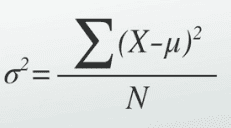# Python variance: How to Calculate Variance in Python

There are mainly two ways of defining the variance. First, you have the variance n that you can use when you have the full set and a variance n-1 when you have the sample. In pure statistics, the variance is the squared deviation of the variable from its mean. It measures the spread of the random data in the set from its mean or median value.

A low value for variance indicates that the data are clustered together and are not spread apart widely. In contrast, the high value would suggest that the data in the given set are much more spread apart from an average value.

A variance is an essential tool in the sciences, where statistical analysis of data is common. It is the square of the standard deviation of the given dataset and is also known as the second central moment of a distribution.

The following formula calculates variance.## Python variance

Python variance() is a built-in function used to calculate the variance from the sample of data (sample is a subset of populated data). Python statistics module provides potent tools which can be used to compute anything related to Statistics. The variance() is one such function. In this blog, we have already seen the Python Statistics mean(), median(), and mode() function.

## Steps to Finding Variance

So let’s break this down into some more logical steps.

1. Find a mean of the set of data.
2. Subtract each number from a mean.
3. Square the result.
5. Divide a result by the total number of numbers in the data set.

### Syntax

The syntax of the variance() function in Python is the following.

`statistics.variance(data, xbar=None)`

If the data has fewer than two values, StatisticsError raises.

### Arguments

#data:

This parameter is required when data is an array of valid Python numbers, including Decimal and Fraction values.

#xbar:

Where xbar is the mean of data, this parameter is optional. The mean is automatically calculated if this parameter is not given(none).

The variance() function is only available and compatible with Python 3.x.

### Example

See the following example.

```# app.py

import statistics

dataset = [21, 19, 11, 21, 19, 46, 29]
output = statistics.variance(dataset)

print(output)
```

See the following output.

```➜  pyt python3 app.py
124.23809523809524
➜  pyt```

## Python variance() with both Arguments

Calculate the mean first and pass it as an argument to the variance() method. See the following code.

```# app.py

import statistics

dataset = [21, 19, 11, 21, 19, 46, 29]
meanValue = statistics.mean(dataset)
output = statistics.variance(dataset, meanValue)

print(output)
```

See the following output.

```➜  pyt python3 app.py
124.23809523809524
➜  pyt```

## Calculate variance() of Fraction

Use Fraction array as an argument.

```# app.py

from decimal import Decimal as D
from statistics import variance

print(variance([D("21.11"), D("19.21"), D("46.21"), D("18.21"), D("29.21"), D("21.06")]))
```

See the following output.

```➜  pyt python3 app.py
114.73775
➜  pyt```

## Compute the Variance in Python using Numpy

In this example, we use the numpy module.

Variance measures how far the set of (random) numbers are spread out from their average value.

In Python language, we can calculate a variance using the numpy module.

With the numpy module, the var() function calculates variance for the given data set. See the following example.

```# app.py

import numpy as np

dataset= [21, 11, 19, 18, 29, 46, 20]

variance= np.var(dataset)

print(variance)```

See the output.

```➜  pyt python3 app.py
108.81632653061224
➜  pyt```

So let’s break down the above code.

We import the numpy module as np. This means that we reference the numpy module with the keyword np.

We then create the variable, dataset, which is equal to [21, 11, 19, 18, 29, 46, 20]

We then get a variance of the dataset by using an np.var() function. So instead of the np.var() function, we specify the variable, the dataset.

We then print out the variance, which in this case, is 108.81632653061224.

So let’s go over the formula for a variance to see if this value is correct.

The formula for variance is, variance= (x-mu)2/n

And this is how you can compute the variance of a data set in Python using the numpy module.

That’s it for this tutorial.

Python mean()

Python mode()

Python median()

Python stddev()

Python sum()

### 1 thought on “Python variance: How to Calculate Variance in Python”

1.You’re confusing my friend(s) by using “variance” to refer to both population variance and sample variance methods. And I agree it is confusing, especially for beginners. I think you can make the tutorial clear by stating statistics.variance() computes *sample variance* and numpy.var() computes *population variance*; using these terms would remove confusion. You show the formula for calculating variance (in pure statistics, i.e. sample variance) then immediately jump into the section for statistics.variance() which uses sample variance, and that probably confuses many readers. Readers skip over the (changing) definitions you give or don’t understand them, so I think it’d be better to explicitly state sample or population variance.

Some things that might flesh out this tutorial some more:
1) statistics.pvariance() can also be used to calculate population variance
2) numpy.var() has a ddof parameter. np.var(dataset,ddof=1) can also be used to calculate sample variance. ddof stands for delta degrees of freedom; it’s the 1 in the N – 1 part of the sample variance formula. I.e. N – ddof.
3) Maybe use the same dataset throughout the tutorial where possible.

Thanks. I hope this comment reaches you.